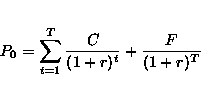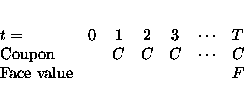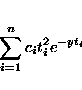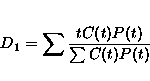BOND PRICING
Wait until calculator button appears. Sometimes You Need To Press Refresh

The price of a bond is the present value of its future cashflows.
If we consider a coupon bond like a US goverment bond (T-Bond),
the cash flows look likeThe current price of the bond iswith discrete compounding,
and with continous compounding.The interest rate is fixed,
which means that the term structure is flat.
Convexity measures the curvature of the approximation done
when using duration.
It is calculated asSimple Duration where
C(t) is the cashflow in period t